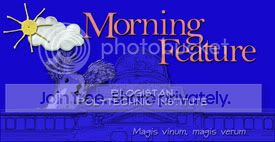Are gods discovered or invented? What about rights? Such questions have no provable answers, partly because we disagree on which gods (if any) or which claimed rights are correct.

But many mathematical questions have provably correct answers. And Mario Livio asks: are those discovered or invented? Is god a mathematician? (More)

Is God a Mathematician? (Non-Cynical Saturday)

This week Morning Feature reviews our summer reading. Thursday we looked at Max Blumenthal’s Republican Gomorrah. Yesterday we considered Andrew Bacevich’s Washington Rules through the historical lens of Edward Miller’s War Plan Orange. Today we conclude with Mario Livio’s Is God a Mathematician?

Mario Livio is an astrophysicist and head of the Office of Public Outreach at the Hubble Space Telescope Institute. He’s also a gifted writer about mathematics, and his latest book – Is God a Mathematician? – explores a question that mathematicians and philosophers have argued for millenia: are mathematical ideas discoveries about the essential nature of the universe, or human inventions like new words in a language?

Why does it matter?

The question may seem trivial, but consider the similar questions that open the introduction. We have argued and even waged wars over whether a claimed divine being or human right exists in nature as ‘discovered’ by prophets or philosophers, or is merely a human invention. To be sure, such arguments usually focus less on the epistemology than the effects of a group declaring that others must recognize and comply with a god or set of rights.

That also happens with mathematics. In 1990, Iraq claimed as a casus belli that Kuwaiti oil wells were “drilled on a slant,” drawing oil from across the border. That is a claim based on geometry as much as international law. Many political controversies have mathematical forms – from the trimester analysis of Roe v. Wade to eligibility age and calculations for Social Security benefits – and political arguments often use mathematical principles such as statistics.

We tacitly assume that the percentage of persons who live past a given age is a fact, and we should accept mathematical facts in the same way and for the same reason we accept gravity: both express an objective reality independent of human desire or opinion. Thus mathematical facts – and by implication the principles and methods by which we derive them – are discoveries and not inventions. Thus we assume … but is that assumption true?

Math’s “unreasonable effectiveness.”

Dr. Livio offers a case for mathematics-as-discovery based on what he calls the “unreasonable effectiveness” of mathematics in describing the universe. Mathematical principles enable predictions of such accuracy that not even selection bias – counting predictions that work and ignoring those that don’t – seems sufficient to explain away. Each of us acts on mathematical predictions thousands of times a day. We flip a switch and current flows to a coffee pot, as predicted by Maxwell’s equations almost 140 years ago. We tip a coffee pot over a mug and the stream flows on a parabolic arc, as predicted by Newton’s equations over 320 years ago.

“Well yes,” you might say, “but Maxwell and Newton used mathematics to describe common observations. We make similar observations, so of course our observations match their equations.”

Dr. Livio calls that active effectiveness, mathematical principles developed and refined to describe and predict the phenomena for which we apply them. But there is also what Dr. Livio calls passive effectiveness, principles that were developed for another application – or simply as abstract exercises with no application in mind – that later provide remarkably effective models of observations in another field. The spatial properties of curved surfaces, non-Euclidean geometry, were at first merely abstract ideas that mathematicians played with from the 16th to 19th centuries. But in the early 20th century, Albert Einstein used those properties to precisely describe the curved space of general relativity.

The “unreasonable [active and passive] effectiveness” of mathematics in describing and predicting real world phenomena suggests that mathematics must be more than mere human invention. Mathematicians must discover things that exist essentially, completely, and consistently in the real universe, right? Well….

Enter Gödel. Exit completeness.

That seemed to work, as Dr. Livio tells the story, until Kurt Gödel came along. A logician, Gödel conclusively proved that no non-trivial logical structure can be both complete and consistent. It must either include axioms that cannot be proved within itself, or have irresolvable logical paradoxes, or both. And as the principles of mathematical systems are derived through logic, Gödel’s theorem of incompleteness must apply to mathematical systems as well.

If the real universe is comprised of mathematical principles – the most extreme version of mathematics-as-discovery, argued by MIT professor Max Tegmark and others – then by Gödel’s theorem the real universe must be incomplete and/or inconsistent. If it is inconsistent, mathematical facts that are true here-and-now may not be true everywhere-and-always, contrary to core assumptions of science. And if the universe is logically incomplete, where would we find the external bits necessary to prove any mathematical principle within it?

Ultimately, Dr. Livio leaves the question unanswered. Or rather, he admits that he cannot prove the answer he proposes: the concepts of mathematics are invented, but the principles that develop and connect those concepts are discovered.

For example, the Greeks invented the concept of prime numbers. Dr. Livio argues that they needn’t have done so, noting that powerful mathematical systems in China and elsewhere did not include the idea of prime numbers. But having invented that concept, the principles of prime numbers – their definition, relationships, and uses – were discovered as the only possible correct expressions of that concept.

So must we yield to mathematical facts as we yield to gravity? Yes, but only to the extent that we agree to value the concepts being measured or described. Hrmm … facts matter, subject to values. That sounds very familiar….

+++++

Happy Saturday!